# 算法：递归，贪心，分治，动态规划

26 Apr 2021

### 递归

#### 斐波那契数列

f(1) = 1, f(2) = 1, f(n)=f(n-1)+f(n-2) ( n>=3 )

``````def fib1(n: Int):Int = {
if (n <= 1) n else fib1(n-1)+fib1(n-2)
//     if (n <= 2) n  定义了递归基，如果小于2的情况终止递归
// 		 fib1(n-1)+fib1(n-2) 递归调用
}
``````

• 时间复杂度：O(2^n)
• 空间复杂度: O(n)
• 递归调用的空间复杂度 = 递归深度 * 每次调用所需的辅助空间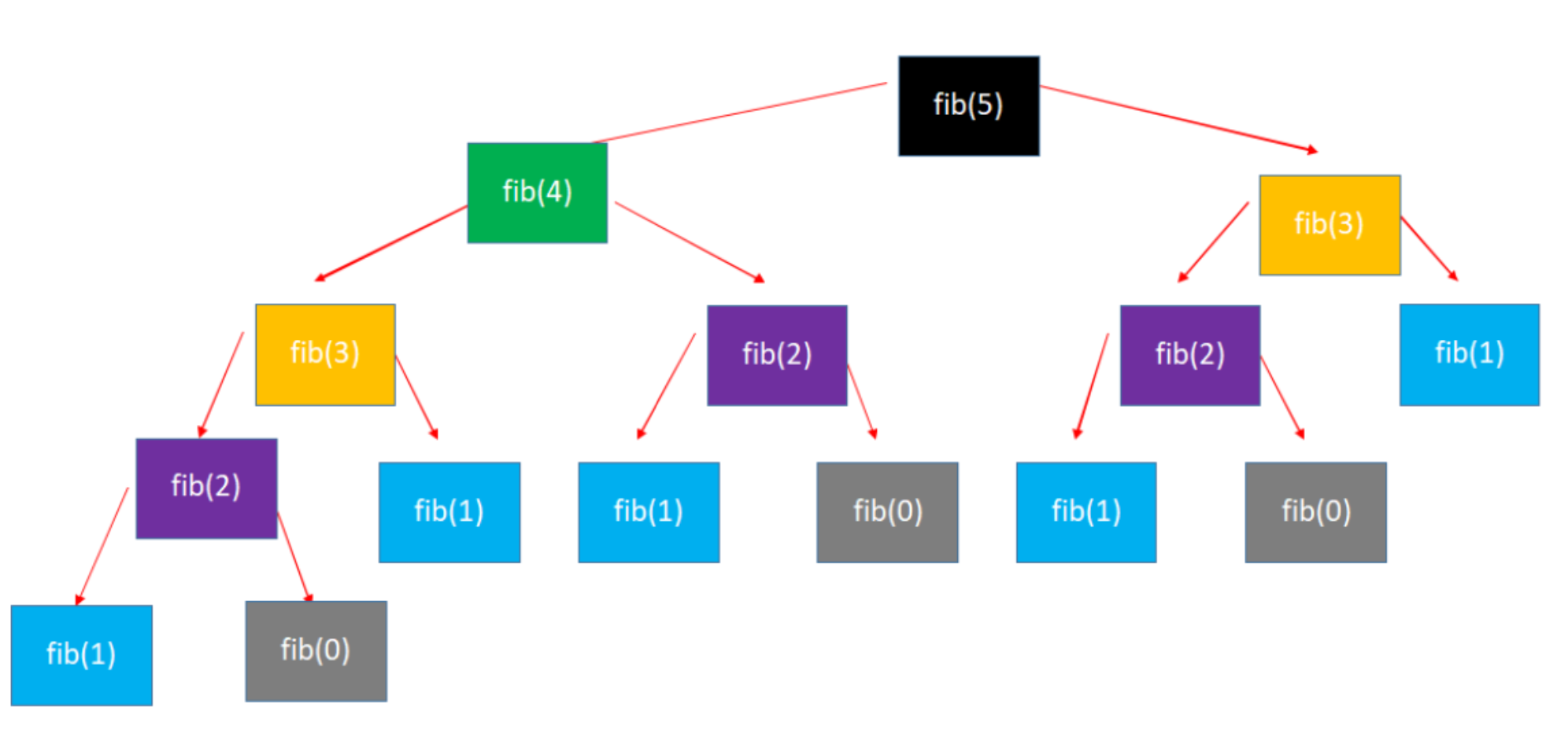``````// 使用数组
def fib2(n: Int):Int = {
// 不需要递推的情况
if (n <= 1) return n

val list = new Array[Int](n+1)
// 递推基
list(1) = 1
for (i <- 2 to n) {
list(i) = list(i-1) + list(i-2)
}

list.last
}
``````

``````// 使用变量
def fib3(n: Int):Int = {
if (n <= 1) return n

var first = 0
var second = 1
for (_ <- 2 to n) {
second = first+second
first = second-first
}
second
}
``````

``````使用递归往往不是为了求得最优解，是为了简化解决问题的思路，代码会更简洁!!

``````

#### 评价算法

##### 事后统计法

``````// java
import java.text.SimpleDateFormat;
import java.util.Date;

public class Times {
private static final SimpleDateFormat fmt = new SimpleDateFormat("HH:mm:ss.SSS");

void execute();
}

name = (name == null) ? "" : ("[ " + name + " ]");
System.out.println(name);
System.out.println("begin : " + fmt.format(new Date()));
long begin = System.currentTimeMillis();
long end = System.currentTimeMillis();
System.out.println("end: " + fmt.format(new Date()));
double delta = (end - begin)/1000.0;
System.out.println("duration: " + delta + " s");
System.out.println("---------------------------------------------");
}
}
``````

• 执行时间取决于硬件以及运行时各种不确定的环境因素(开启很多软件与没开启很多软件)

• 必须编写测试代码

• 测试数据的选择比较难保证公正性(有些算法数据量小可能快，大了就不行，有些相反)

• 正确性、可读性、健壮性(对不合理输入的反应能力和处理能力)
• 时间复杂度(Time complexity):估算程序指令的执行次数(执行时间)
• 空间复杂度(Space complexity):估算所需占用的存储空间
##### 大O表示法(Big O)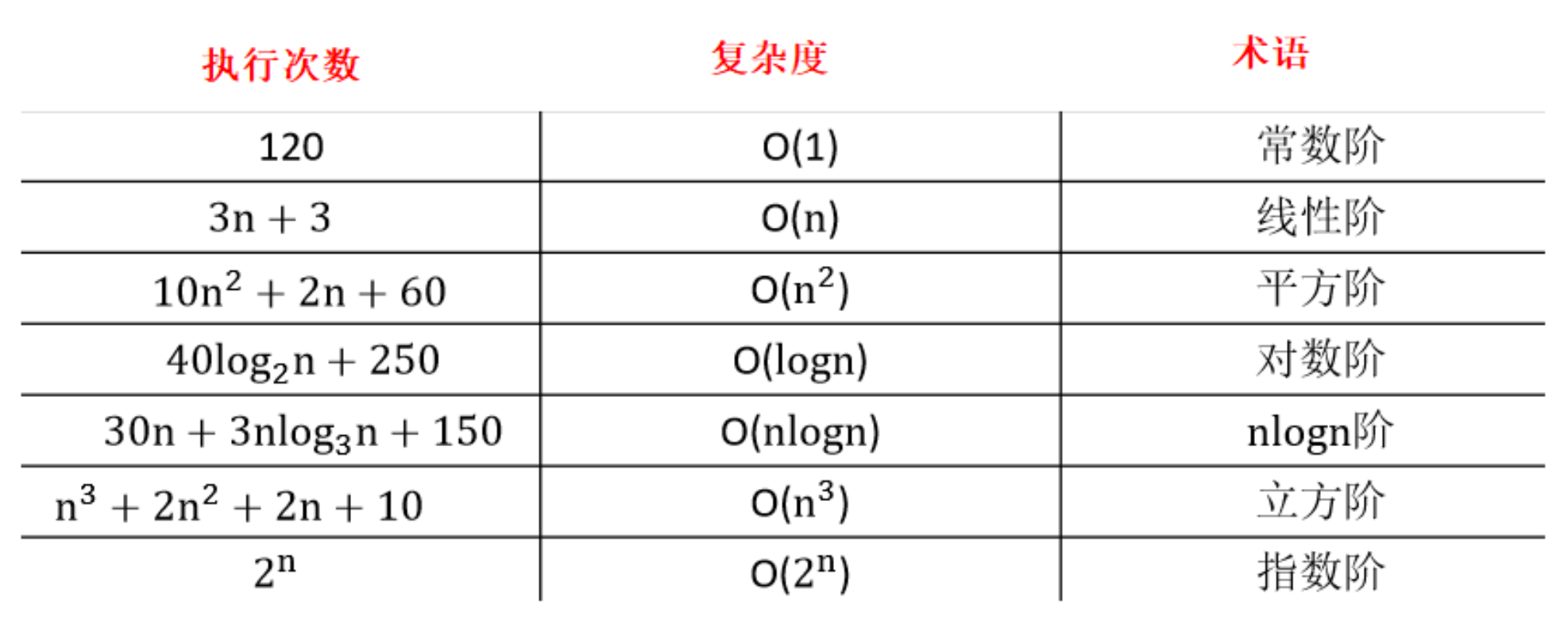`````` O(1) < O(logn) < O(n) < O(nlogn) < O(n2) < O(n3) < O(2n) < O(n!) < O(nn)
``````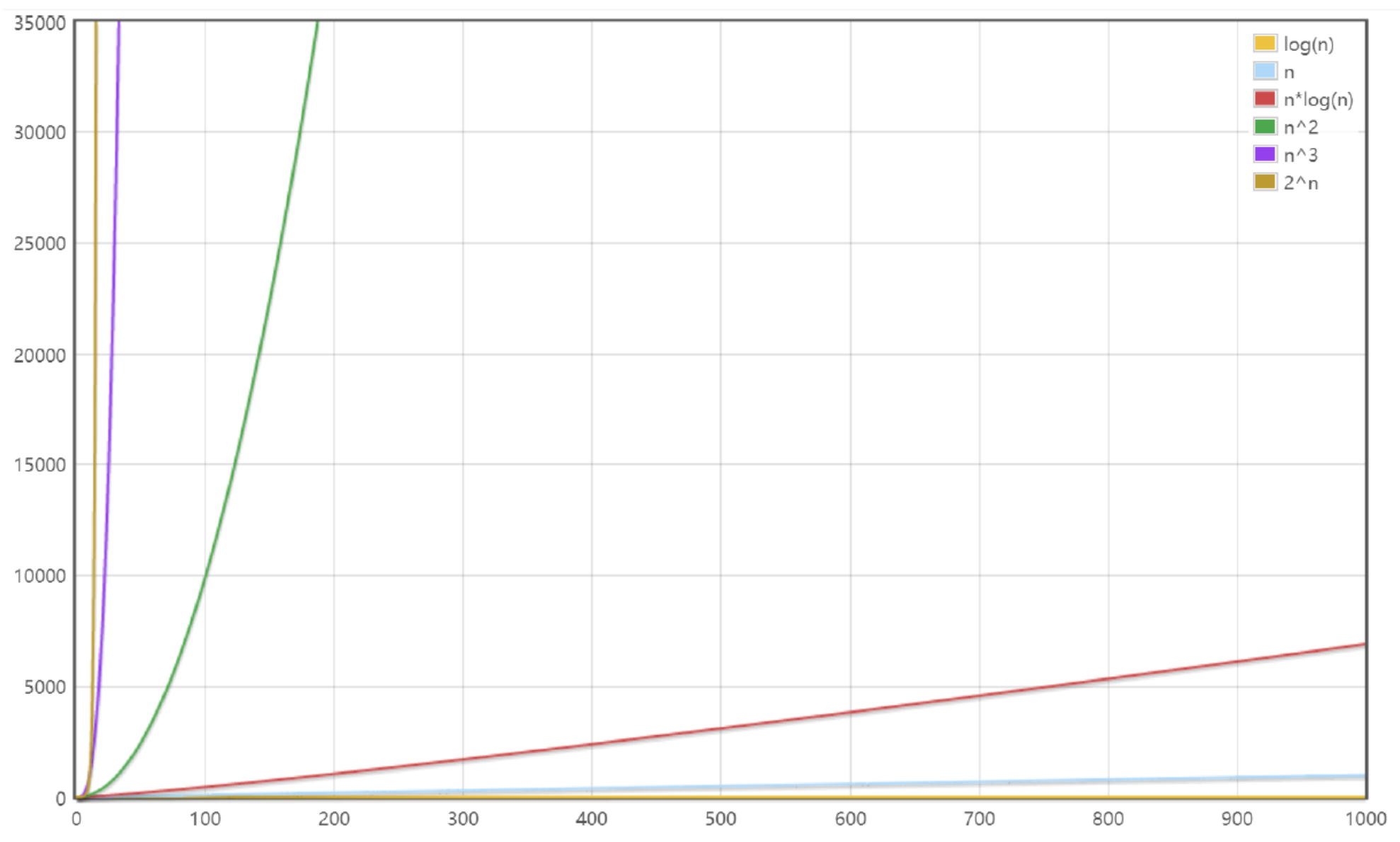``````def fib1(n: Int):Int = {
if (n <= 1) n else fib1(n-1)+fib1(n-2)
}
// 时间复杂度：O(2^n)
// 空间复杂度：O(n)

def fib2(n: Int):Int = {
// 不需要递推的情况
if (n <= 1) return n

val list = new Array[Int](n+1)
// 递推基
list(1) = 1
for (i <- 2 to n) {
list(i) = list(i-1) + list(i-2)
}

list.last
}
// 时间复杂度：O(n)
// 空间复杂度：O(n)

def fib3(n: Int):Int = {
if (n <= 1) return n

var first = 0
var second = 1
for (_ <- 2 to n) {
second = first+second
first = second-first
}
second
}
// 时间复杂度：O(n)
// 空间复杂度：O(1)
``````

• 尽可能少的执行时间
• 尽可能少的存储空间
• 根据情况，可以空间换时间 时间换空间

#### 递归基本思想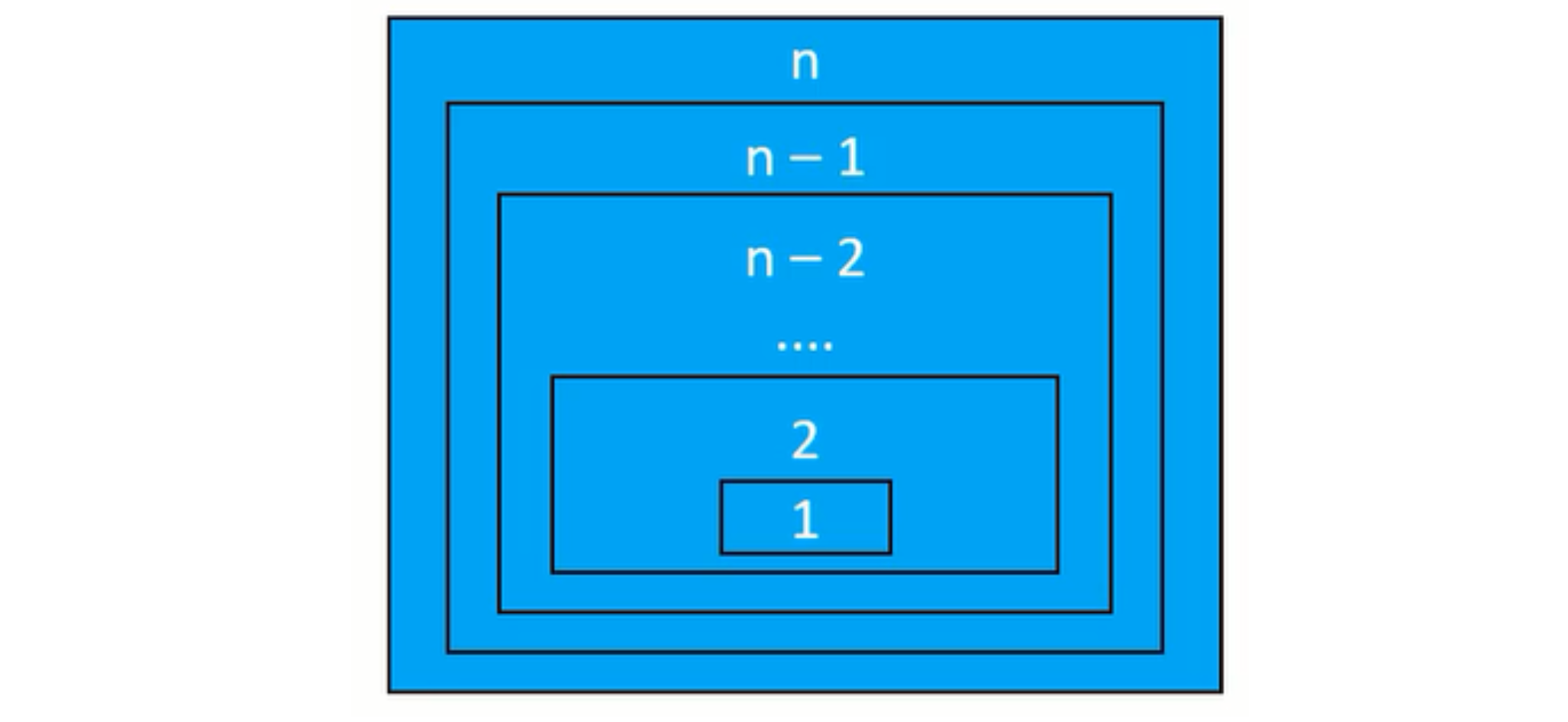• 拆分问题
• 把规模大的问题编程规模较小的同类型问题
• 规模较小的问题又不断变成规模更小的问题
• 规模小到一定程度就可以直接得到结果
• 求解
• 由最小规模问题的解推导出较大规模问题的解
• 由较大规模问题的解不断推导出规模更大问题的解
• 最后推导出原来问题的解

#### 递归使用步骤与技巧

• 确定函数的功能：第一步先不要思考函数里面代码逻辑如何实现，先搞清楚这个函数的目的，完成什么事情?

• 确定子问题与原问题的关系：找到 f(n) 与 f(n – 1) 的关系

• 明确递归基(边界条件)

• 递归的过程中，问题的规模在不断减小，当问题缩减到一定程度便可以直接得出它的解

• 寻找递归基，等价于:问题规模小到什么程度可以直接得出解?

#### 跳台阶

``````object StairDemo {
def main(args: Array[String]): Unit = {
for (i <- 1 to 5) {
println(s"\$i - \${stair(i)}")
}
}

/**
* 楼梯有 n 阶台阶，上楼可以一步上 1 阶，
* 可以一步上 2 阶，走完 n 阶台阶共有多少种不同的走法?
* @param n
* @return
*/
def stair(n: Int):Int = {
/**
* 假设第一次走 1 阶，还剩 n – 1 阶，共 f(n – 1) 种走法
* 假设第一次走 2 阶，还剩 n – 2 阶，共 f(n – 2)种走法
* 所以f(n)=f(n-1)+f(n-2)
* f(1) = 1         1
* f(2) = (1,1) , 2;     2
* f(3) = f(1)+f(2);       3
* f(4) = f(n-1)+f(n-2);     5
*/
if (n<=2) n else stair(n-1)+stair(n-2)
}
}
``````

#### 汉诺塔(Hanoi)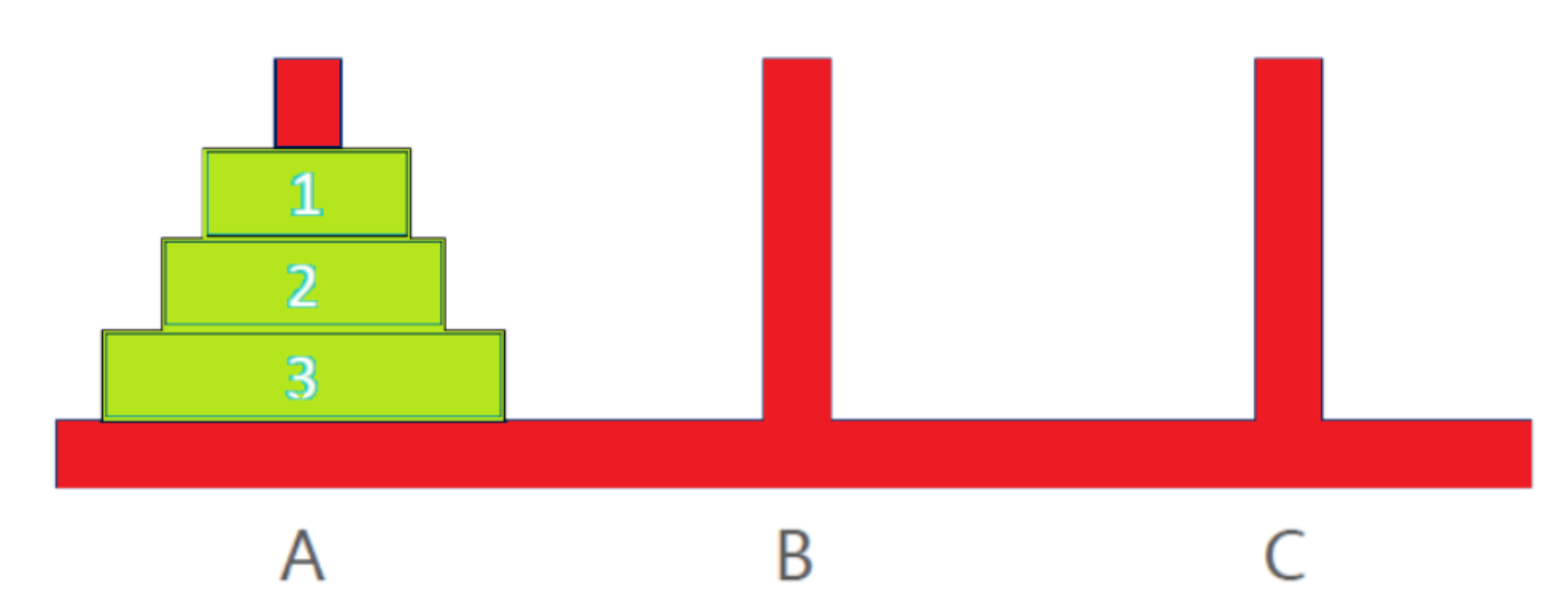• 每次只能移动 1个盘子
• 大盘子必须放在小盘子下面(挪动过程中也是如此)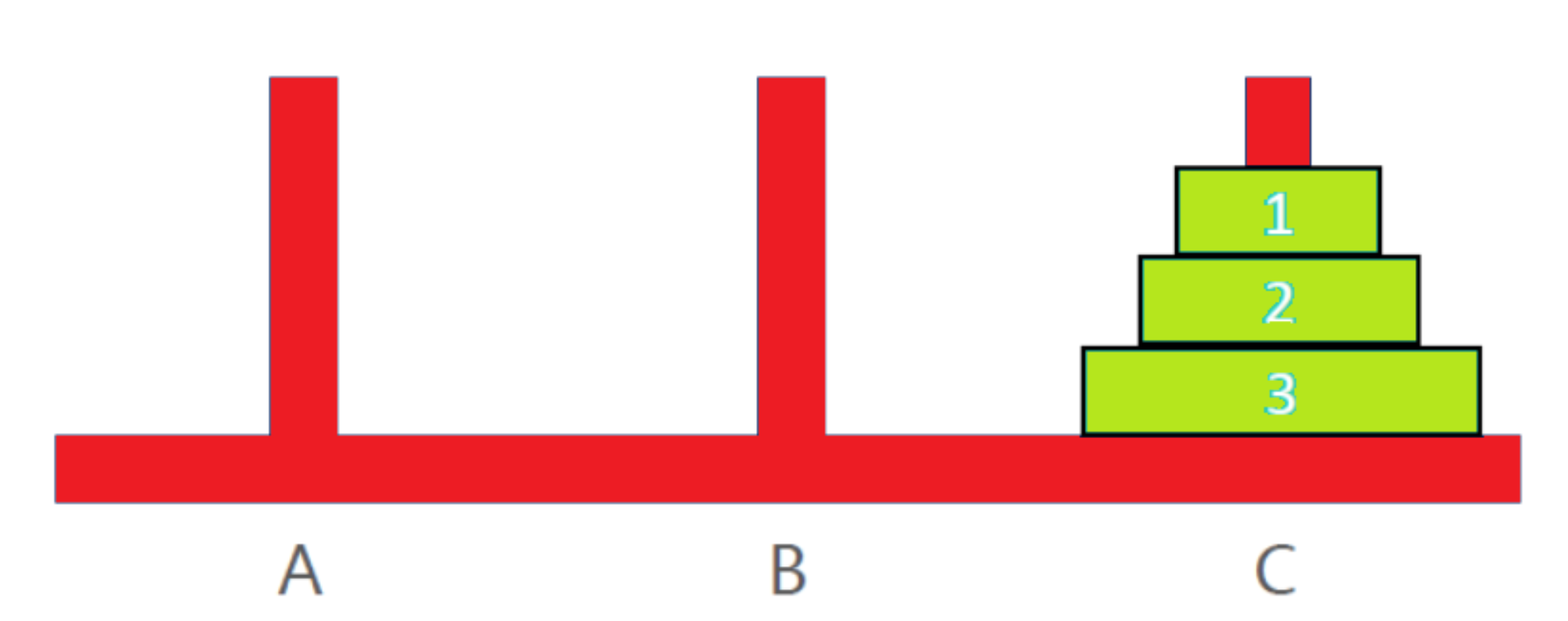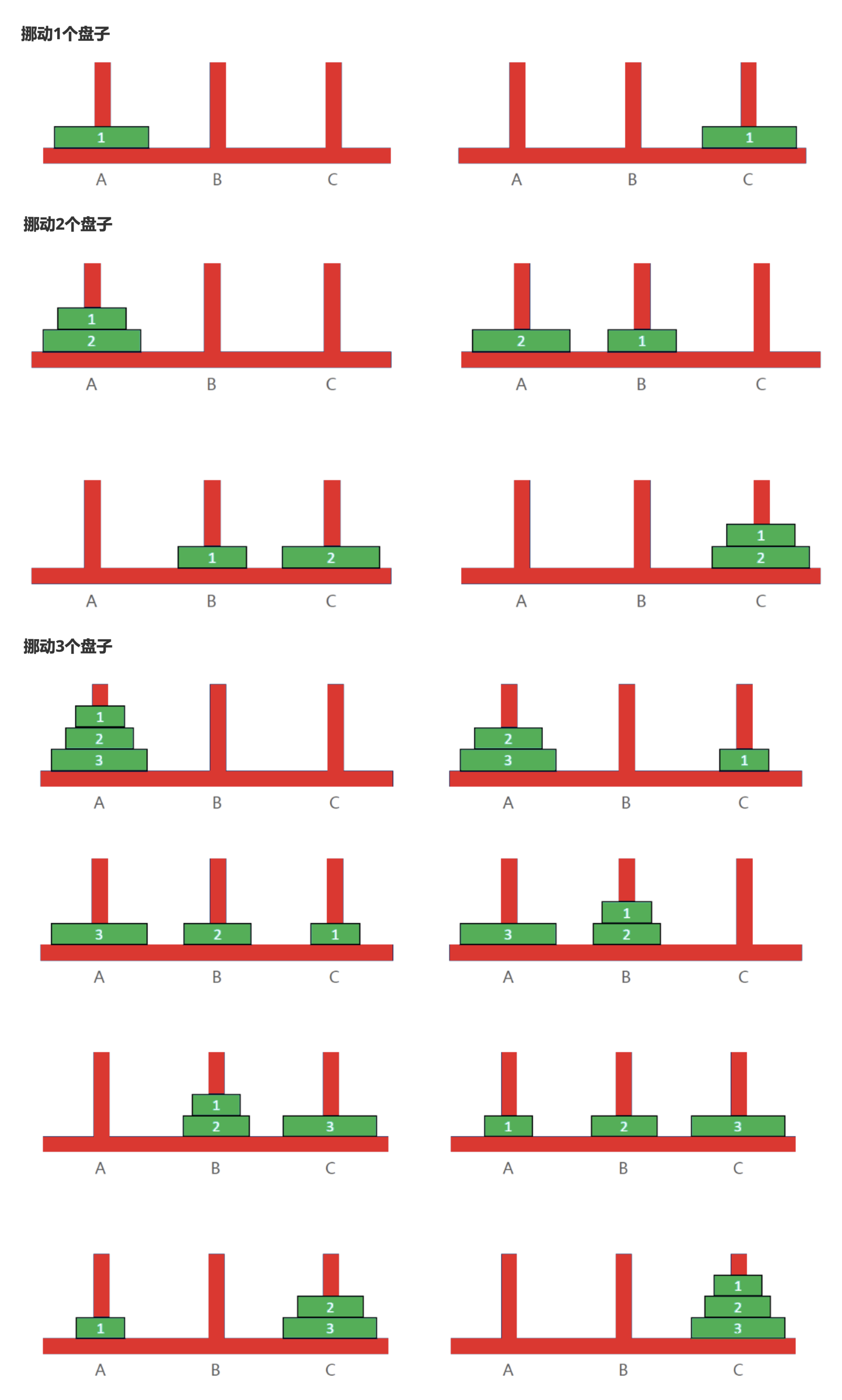• 如果只有一个盘子，n==1时，直接将盘子从A移动到C;
• 如果不止一个盘子，n>=2时，其实可以分为三步
• 将n-1个盘子从A移动到B
• 将编号为n的盘子从A移动到C
• 将n-1个盘子从B移动到C

``````/**
* 只打印步骤
* @param n
* @param a
* @param b
* @param c
*/
def moveHanoi(n: Int, a: String, b: String, c: String):Unit = {
// 将n-1个盘子从A移动到B
// 将编号为n的盘子从A移动到C
// 将n-1个盘子从B移动到C

if (n > 1) {
moveHanoi(n-1, a, c, b)
println(s"move \$n from \$a to \$c")
moveHanoi(n-1, b, a, c)
}
else {
println(s"move \$n from \$a to \$c")
}
/**
* 复杂度分析
* 时间复杂度:O(2^n)
* 空间复杂度:O(n)
**/
}
/**
* 打印步骤，并统计步数
* @param n
* @param a
* @param b
* @param c
* @param prevCount 初始值，会在递归中累加
* @return 当前循环的结果
*/
def moveHanoi(n: Int, a: String, b: String, c: String, prevCount: Int): Int = {
// 将n-1个盘子从A移动到B
// 将编号为n的盘子从A移动到C
// 将n-1个盘子从B移动到C
if (n > 1) {
val count = moveHanoi(n-1, a, c, b, prevCount) + 1
println(s"\$count : move \$n from \$a to \$c")
moveHanoi(n-1, b, a, c, count)
}
else {
val count = prevCount+1
println(s"\$count : move \$n from \$a to \$c")
count
}
}
``````

• 自己使用栈
• 考虑重复利用一组相同的变量

``````// 使用栈来实现，去掉递归掉用
def moveHanoi2(n: Int, a: String, b: String, c: String): Unit = {
// 将n-1个盘子从A移动到B
// 将编号为n的盘子从A移动到C
// 将n-1个盘子从B移动到C

// Boolean, Int, String, String, String
// isPrint, Int, from, middle, to
val stack = new mutable.ArrayStack[(Boolean, Int, String, String, String)]()

/**
* 使用一个stack来存需要处理的状态，遇到print就打印，遇到其他就展开后重新放回
*/
if (n > 1) {
stack.push((false, n-1, b, a, c))
stack.push((true, n, a, b, c))
stack.push((false, n-1, a, c, b))
} else {
stack.push((true, n, a, b, c))
}

while (stack.nonEmpty) {
val (isPrint, nn, from, middle, to) = stack.pop()
if (isPrint) {
println(s"move \$nn from \$from to \$to")
} else if (nn > 1) {
stack.push((false, nn-1, middle, from, to))
stack.push((true, nn, from, middle, to))
stack.push((false, nn-1, from, to, middle))
} else {
stack.push((true, nn, from, middle, to))
}
}
}
``````

### 贪心

#### 海盗运货-最优装载问题

``````/**
1、选择重量为 2 的古董，剩重量 28
2、选择重量为 3 的古董，剩重量 25
3、选择重量为 4 的古董，剩重量 21
4、选择重量为 5 的古董，剩重量 16
5、选择重量为 7 的古董，剩重量 9

*/
def piratePackage():Int = {
val capacity = 30
var goods = List(3,5,4,10,7,14,2,11)
// 按照重量升序
goods = goods.sorted

var res = capacity
var index = 0
while (res > goods(index)) {
res -= goods(index)
index+=1
}
index
}
``````

#### 零钱兑换

• 选择 25 分的硬币，剩 16 分
• 选择 5 分的硬币，剩 11 分
• 选择 5 分的硬币，剩 6 分
• 选择 5 分的硬币，剩 1 分
• 选择 1 分的硬币

``````/**
* 零钱兑换
* 假设有 25 分、5 分、1 分的硬币，现要找给客户 41 分的零钱，如何办到硬币个数最少?
*/
def getCoins(money: Int, faces: List[Int]): Unit = {
val total = money
val sortedFaces = faces.sorted.reverse

var count = 0
var res = total
var index = 0
// 假设肯定能兑换成功
while (res != 0) {
// 如果可以兑换就兑换，不然就换小面额，继续循环
if (res >= sortedFaces(index)) {
res -= sortedFaces(index)
count+=1
println(sortedFaces(index))
} else {
index+=1
}
}

println(s"coin count: \$count")
}
``````

• 优点

• 简单、高效、不需要穷举所有可能，通常作为其他算法的辅助算法来使用
• 缺点

• 目光短浅，不从整体上考虑其他可能，每一步只采取局部最优解，不会对比其他可能性，因此贪心很少情况能获得最优解。

#### 0-1背包问题

• 在保证总重量不超过 W 的前提下，将哪几件物品装入背包，可以使得背包的总价值最大?
• 注意:每个物品只有 1 件，也就是每个物品只能选择 0 件或者 1 件，因此这类问题也被称为 0-1背包问题

• 价值主导:优先选择价值最高的物品放进背包
• 重量主导:优先选择重量最轻的物品放进背包
• 价值密度主导:优先选择价值密度最高的物品放进背包(价值密度 = 价值 ÷ 重量)

``````case class Item(weight: Double, value: Double) {
val dense:Double = value/weight

override def toString: String = s"item(\$weight, \$value, \$dense)"
}
object PackSackDemo {
def main(args: Array[String]): Unit = {
val items = List(
Item(35, 10),
Item(30, 40),
Item(60, 30),
Item(50, 50),
Item(40, 35),
Item(10, 40),
Item(25, 30)
)

println("value priority")
packSackMethod[Double](items, e=>{-e.value})
println()
println("weight priority")
packSackMethod(items, e=>{e.weight})
println()
println("dense priority")
packSackMethod(items, e=>{-e.dense})

/**
* value priority
* selected items List(item(50.0, 50.0, 1.0), item(30.0, 40.0, 1.3333333333333333), item(10.0, 40.0, 4.0), item(40.0, 35.0, 0.875))
* total value : 165.0
* res : 20
*
* weight priority
* selected items List(item(10.0, 40.0, 4.0), item(25.0, 30.0, 1.2), item(30.0, 40.0, 1.3333333333333333), item(35.0, 10.0, 0.2857142857142857), item(40.0, 35.0, 0.875))
* total value : 155.0
* res : 10
*
* dense priority
* selected items List(item(10.0, 40.0, 4.0), item(30.0, 40.0, 1.3333333333333333), item(25.0, 30.0, 1.2), item(50.0, 50.0, 1.0), item(35.0, 10.0, 0.2857142857142857))
* total value : 170.0
* res : 0
*/
}

/**
* 根据排序选择器进行排序，并从左到右开始装包
* @param items 所有物品
* @param comp  排序选择器
* @tparam B double
*/
//<:Double Double 及其子类
//>:Double Double 的父类
def packSackMethod[B<:Double](items: List[Item], comp: Item=>B): Unit = {
implicit val ord = new Ordering[B] {
override def compare(x: B, y: B): Int = x.compareTo(y)
}

val capacity = 150  //total
var res = capacity    //current capacity
val sortedItems = items.sortBy(comp)

// cache selected items
val arrayBuffer = new ArrayBuffer[Item]()
for (it <- sortedItems) {
if (it.weight.intValue() <= res) {
res -= it.weight.intValue()
arrayBuffer += it
}
}

println(s"selected items \${arrayBuffer.toList}")
println(s"total value : \${arrayBuffer.map(_.value).sum}")
println(s"res : \$res")
}
}
``````

### 分治

1、将原问题分解成若干个规模较小的子问题(子问题和原问题的结构一样，只是规模不一样)

2、子问题又不断分解成规模更小的子问题，直到不能再分解(直到可以轻易计算出子问题的解)

3、利用子问题的解推导出原问题的解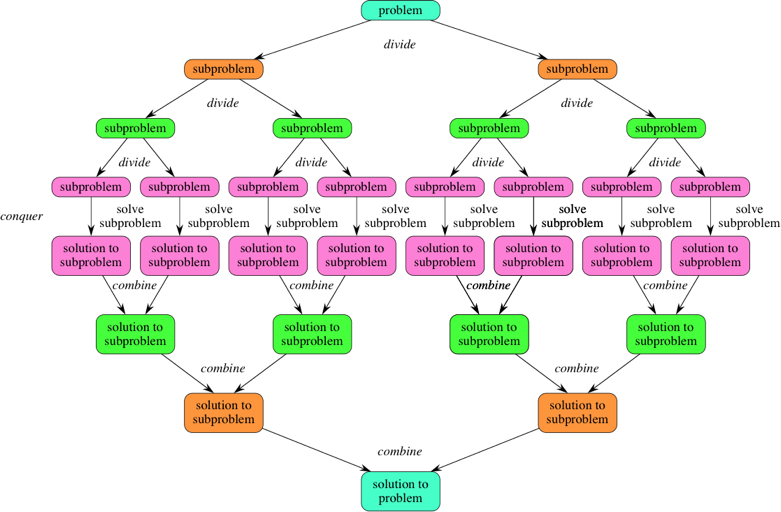#### 快速排序(Quick Sort)

Quick Sort分析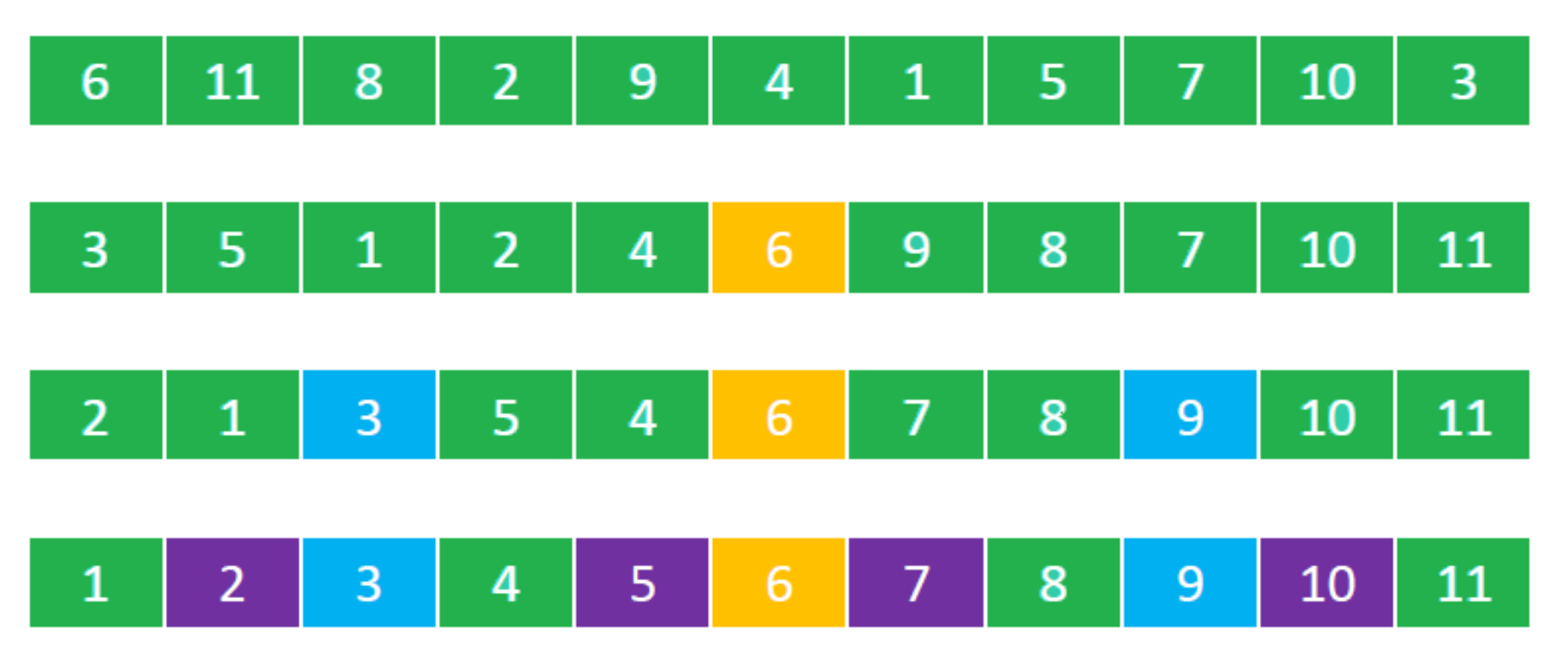• 第一步： 从数组中选择一个轴点元素(Pivot element)，一般选择0位置元素为轴点元素
• 第二步
• 利用Pivot将数组分割成2个子序列
• 将小于 Pivot的元素放在Pivot前面(左侧) ，将大于 Pivot的元素放在Pivot后面(右侧) ，等于Pivot的元素放哪边都可以(暂定放在左边)
• 第三步：对子数组进行第一步，第二步操作，直到不能再分割(子数组中只有一个元素)

Quick Sort的本质: 不断地将每一个元素都转换成轴点元素!!

``````public class JavaQuickSort {
private Integer[] arr;

public static void main(String[] args) {
Integer[] array = new Integer[]{6, 2, 1, 5,6, 8,9};

JavaQuickSort js = new JavaQuickSort();
js.sort(array);
}

public void sort(final Integer[] array) {
arr = array;

// 打印排序前的数据
for (int i=0; i< arr.length; i++) {
System.out.print(arr[i]+"_");
}
System.out.println();

// 排序并打印排序后的数据
sort(0, array.length);
for (int i=0; i< arr.length; i++) {
System.out.print(arr[i]+"_");
}
System.out.println();

}

/**
* 快排实现
* @param start  开始排序的的下标
* @param end   结束排序的的下标
*/
public void sort(Integer start, Integer end) {
if (end-start < 2) return;

Integer pivot = getPivotIndex(start, end);

sort(start, pivot);
sort(pivot+1, end);
}

/**
* find define pivotElement and divide arr with pivot, return new pivot index
* @param start 开始排序的的下标
* @param end   结束排序的的下标
* @return pivot
*/
private Integer getPivotIndex(Integer start, Integer end) {
Integer pivotElement = arr[start];
end--; //point to the last index

while (start < end) {
// 小于pivot的数据往左挪
while (start<end) {
if(myCompare(pivotElement, arr[end]) < 0) {
end--;
} else {
arr[start] = arr[end];
start++;
break;
}
}
// 大于pivot的数据往右挪
while (start<end) {
if(myCompare(arr[start], pivotElement) < 0) {
start++;
} else {
arr[end] = arr[start];
end--;
break;
}
}
}

arr[start] = pivotElement;

return start;
}

// 定义比较方法，方便修改排序规则
public int myCompare(Integer t1, Integer t2) {
return t1.compareTo(t2);
}
}
``````
• 时间复杂度
• 最坏情况:• 最好情况: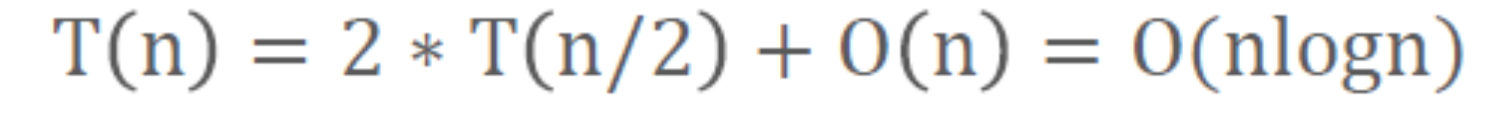• 空间复杂度: 由于递归调用，每次类似折半效果所以空间复杂度是O(logn)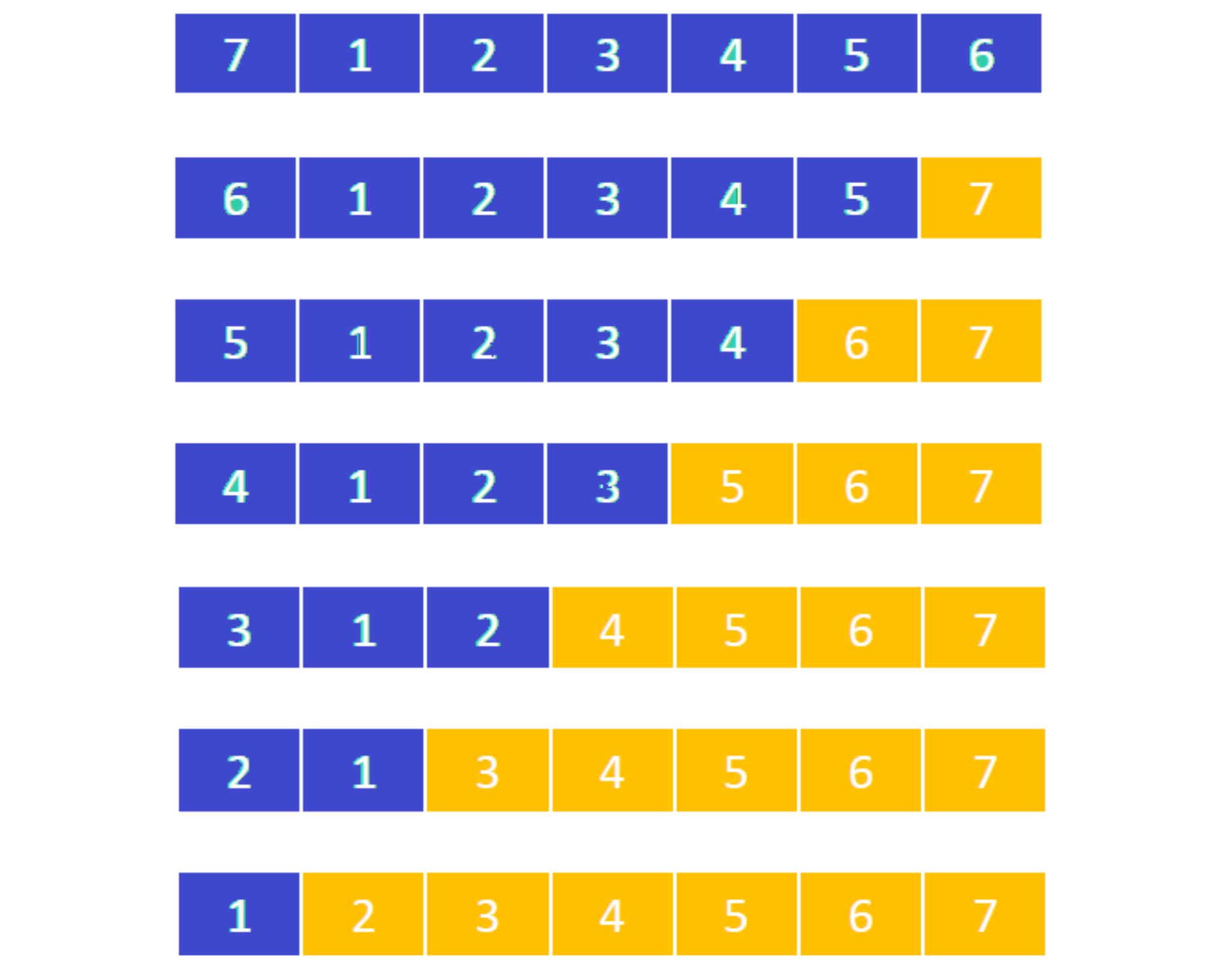``````随机挑选一个pivot，而不是每次都选第一个元素
``````

#### 排序算法的稳定性

``````对于排序算法还有一个评价指标就是稳定性!!

``````

`````` 如果a=b
排序前:5, 1, 2𝑎, 4, 7, 2𝑏
稳定的排序: 1,2𝑎,2𝑏 ,4,5,7
不稳定的排序:1, 2𝑏 , 2𝑎, 4, 5, 7
``````

### 动态规划

• 递归(自顶向下，出现了重叠子问题)
• 递推(自底向上，循环)

``````Dynamic Programming is a method for solving a complex problem by breaking it down into a
collection of simpler subproblems, solving each of those subproblems just once, and
storing their solutions.
``````

``````* 最优化问题(最优子结构问题):通过求解子问题的最优解，可以获得原问题的最优解
* 无后效性
``````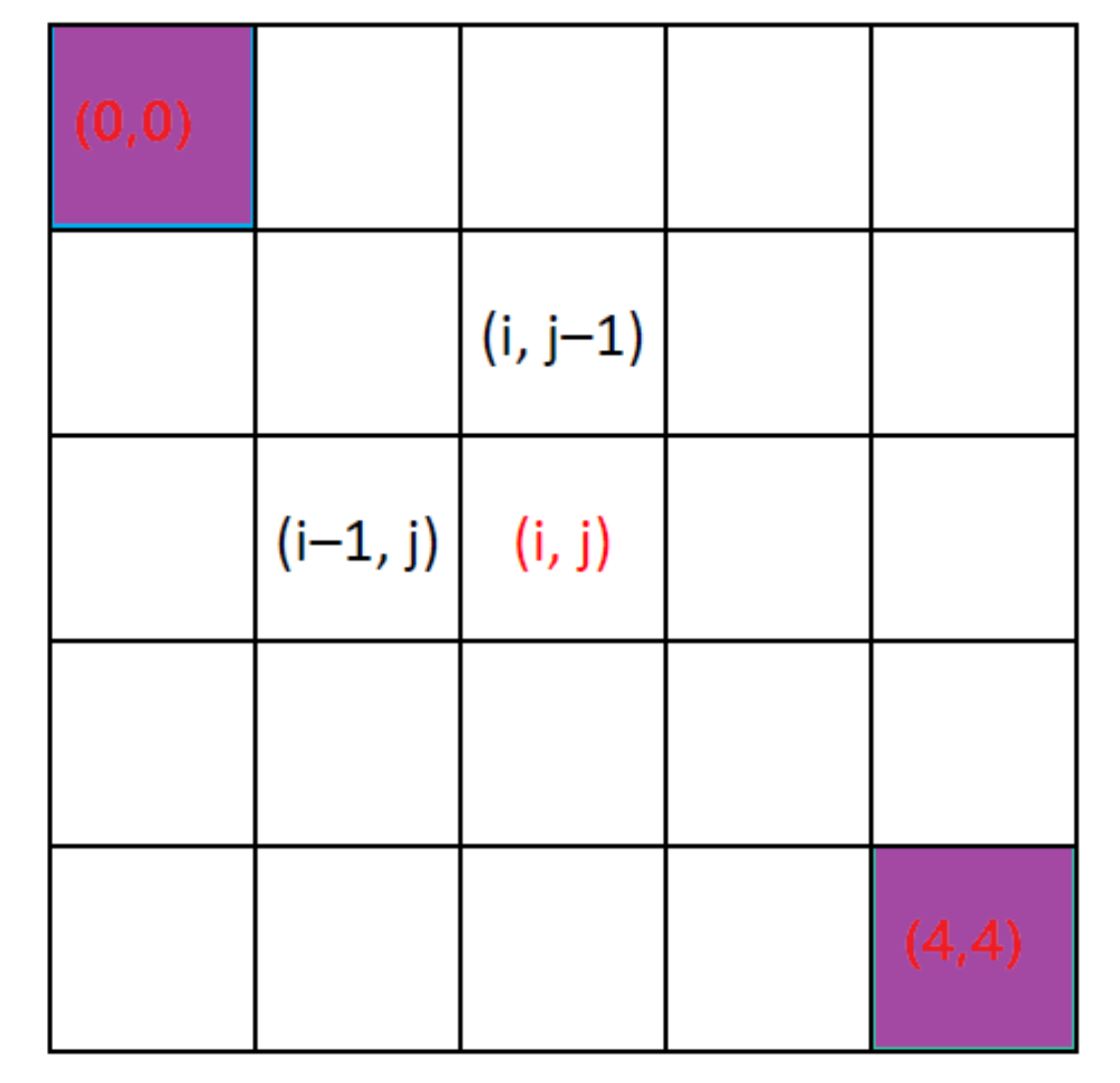• 从起点(0, 0)走到终点(4, 4)一共有多少种走法?只能向右、向下走
• 假设 dp(i, j) 是从(0, 0)走到(i, j)的走法
• dp(i, 0) = dp(0, j) = 1
• dp(i, j) = dp(i, j – 1) + dp(i – 1, j)• dp(i, j) 下一步要怎么走，还要关心上一步是怎么来的
• 还需要考虑 dp(i, j – 1)、dp(i – 1, j) 是怎么来的

#### 实现步骤

• 定义状态：状态指的是原问题，子问题的解，例如dp(i)
• 设定初始状态：问题的边界，比如设置dp(0)的值
• 确定状态转移方程：如确定 dp(i) 和 dp(i – 1) 的关系

#### 零钱兑换

• 定义状态 dp(n):凑到 n 分需要的最少硬币个数

• 设定初始状态

dp(25)=dp(20)=dp(5)=dp(1)=1

• 确定状态转移方程

• 如果第 1 次选择了 25 分的硬币，那么 dp(n) = dp(n – 25) + 1
• 如果第 1 次选择了 20 分的硬币，那么 dp(n) = dp(n – 20) + 1
• 如果第 1 次选择了 5 分的硬币，那么 dp(n) = dp(n – 5) + 1
• 如果第 1 次选择了 1 分的硬币，那么 dp(n) = dp(n – 1) + 1
• 所以 dp(n) = min { dp(n – 25), dp(n – 20), dp(n – 5), dp(n – 1) } + 1
``````//递归(自顶向下，出现了重叠子问题)
def coinChange1(n: Int):Int = {
// 边界状态
if (n < 1) return Int.MaxValue
// 设定初始状态
if(List(1, 5, 20, 25).contains(n)) return 1

// 状态转移方程
List(
coinChange1(n-1),
coinChange1(n-5),
coinChange1(n-20),
coinChange1(n-25)
).min + 1
}
``````

``````//  递推(自底向上，循环)
def coinChange2(n: Int):Int = {
if (n < 1) return Int.MaxValue
if(List(1, 5, 20, 25).contains(n)) return 1

val dp = Array.fill(n+1)(Int.MaxValue)
dp(1) = 1
dp(5) = 1
dp(20) = 1
dp(25) = 1

for (i <- 1 to n) {
if (i > 25) {
dp(i) = List(dp(i-1), dp(i-5), dp(i-20), dp(i-25)).min+1
} else if (i > 20) {
dp(i) = List(dp(i-1), dp(i-5), dp(i-20)).min+1
} else if (i > 5) {
dp(i) = List(dp(i-1), dp(i-5)).min+1
} else if (i > 1) {
dp(i) = dp(i-1)+1
}
}
dp(n)
}
``````

``````/**
* 动态规划实现零钱兑换
* @param amount 需要兑换的总额
* @param coins 币的类型
* @return 最小币数，如果不存在就返回-1
*/
def coinChange4(amount: Int, coins: List[Int]):Int = {
if (amount < 1 || coins == null || coins.isEmpty) return -1

val dp = Array.fill(amount+1)(0)

for (i <- 1 to amount) {
var min = Int.MaxValue

coins.foreach { face =>
if (i >= face && dp(i-face) >= 0) {
min = Math.min(dp(i-face), min)
}
}

if (min == Int.MaxValue) {
dp(i) = -1
} else {
dp(i) = min+1
}
}
dp(amount)
}
``````

``````–2、1、–3、4、–1、2、1、–5、4 的最大连续子序列和是 4 + (–1) + 2 + 1 = 6
``````
• 定义状态

dp(i) 是以 nums[i] 结尾的最大连续子序列和(nums是整个序列)

• 初始状态 dp(0)=nums

• 状态转移方程

``````如果以 nums –2 结尾，则最大连续子序列是 –2，所以 dp(0) = –2
如果以 nums 1 结尾，则最大连续子序列是 1，所以 dp(1) = 1
如果以 nums –3 结尾，则最大连续子序列是 1、–3，所以 dp(2) = dp(1) + (–3) = –2
如果以 nums 4 结尾，则最大连续子序列是 4，所以 dp(3) = 4
如果以 nums –1 结尾，则最大连续子序列是 4、–1，所以 dp(4) = dp(3) + (–1) = 3
如果以 nums 2 结尾，则最大连续子序列是 4、–1、2，所以 dp(5) = dp(4) + 2 = 5
如果以 nums 1 结尾，则最大连续子序列是 4、–1、2、1，所以 dp(6) = dp(5) + 1 = 6
如果以 nums –5 结尾，则最大连续子序列是 4、–1、2、1、–5，所以 dp(7) = dp(6) + (–5) = 1
如果以 nums 4 结尾，则最大连续子序列是 4、–1、2、1、–5、4，所以 dp(8) = dp(7) + 4 = 5
``````
• 如果 dp(i – 1) ≤ 0，那么 dp(i) = nums[i]
• 如果 dp(i – 1) > 0，那么 dp(i) = dp(i – 1) + nums[i]

``````最大连续子序列和是所有 dp(i) 中的最大值 max { dp(i) }，i ∈ [0, nums.length)
``````
``````def maxSubSequence2(list: List[Int]): Int = {
/**
* dp(i) 是以 nums[i] 结尾的最大连续子序列和(nums是整个序列)
* dp(0) = nums
* 如果 dp(i – 1) ≤ 0，那么 dp(i) = nums[i]
* 如果 dp(i – 1) > 0，那么 dp(i) = dp(i – 1) + nums[i]
*
* dp(i) = 最大连续子序列和是所有 dp(i) 中的最大值 max { dp(i) }，i ∈ [0, nums.length)
*/

if (list == null || list.isEmpty) return 0

val dp = new Array[Int](list.size)

for (i <- 1 until list.size) {
dp(i) = list(i) + (if(dp(i-1)<=0) 0 else dp(i-1))
}

dp.max
}
``````

``````// 使用变量代替数组
def maxSubSequence3(list: List[Int]): Int = {
/**
* dp(i) 是以 nums[i] 结尾的最大连续子序列和(nums是整个序列)
* dp(0) = nums
* 如果 dp(i – 1) ≤ 0，那么 dp(i) = nums[i]
* 如果 dp(i – 1) > 0，那么 dp(i) = dp(i – 1) + nums[i]
*
* dp(i) = 最大连续子序列和是所有 dp(i) 中的最大值 max { dp(i) }，i ∈ [0, nums.length)
*/

if (list == null || list.isEmpty) return 0

var max = lastSum
for (i <- 1 until list.size) {
lastSum = list(i) + (if(lastSum<=0) 0 else lastSum)
max = Math.max(max, lastSum)
}

max
}
``````

#### 最长公共子序列(Longest Common Subsequence，LCS)

[1, 3, 5, 9, 10] 和 [1, 4, 9, 10] 的最长公共子序列是 [1, 9, 10]，长度为 3

i ∈ [0, nums1.length]

j ∈ [0, nums2.length]

• 定义状态方程

dp(i, j) 是 [nums1 前 i 个元素] 与[nums2 前 j 个元素] 的最长公共子序列长度

• 定义初始值

dp(i, 0)、dp(0, j) 初始值均为 0

• 定义状态转移方程

• 假设 nums1[i – 1] = nums2[j – 1]，那么 dp(i, j) = dp(i – 1, j – 1) + 1

• 假设 nums1[i – 1] ≠ nums2[j – 1]，那么 dp(i, j) = max { dp(i – 1, j), dp(i, j – 1) }

``````// 二维数组实现
def commonNumsSubsequence2(list1: List[Int], list2: List[Int]):Unit = {
/**
* 1. 状态函数
* dp(i)(j) 表示list1的前i个元素 和 list2的前j个元素的最长子序列长度
*
* 2. 初始状态
* dp(i)(0) = 0
* dp(0)(j) = 0
*
* 3. 状态转移函数
* 假设 list1[i – 1] = list2[j – 1]，那么 dp(i, j) = dp(i – 1, j – 1) + 1
* 假设 list1[i – 1] ≠ list2[j – 1]，那么 dp(i, j) = max { dp(i – 1, j), dp(i, j – 1) }
*/

if (list1 == null || list2 == null || list1.isEmpty || list2.isEmpty) return 0

val dp = Array.ofDim[Int](list1.size+1, list2.size+1)

for (i <- 1 to list1.size; j <- 1 to list2.size) {
if (list1(i-1) == list2(j-1)) {
dp(i)(j) = dp(i-1)(j-1) + 1
} else {
dp(i)(j) = Math.max(dp(i-1)(j), dp(i)(j-1))
}
}

dp.last.last
}
``````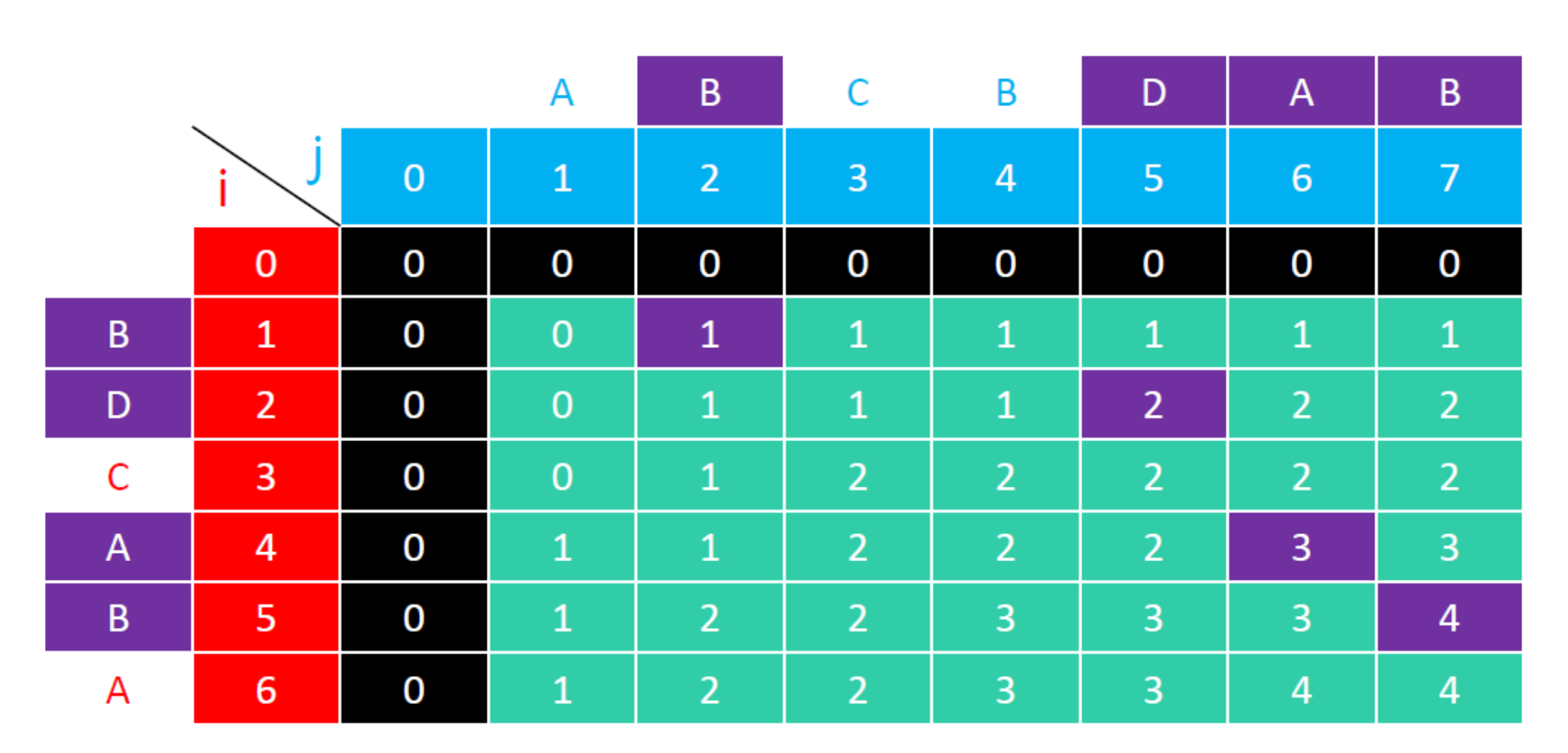``````// 一维数组实现
def commonNumsSubsequence3(list1: List[Int], list2: List[Int]):Unit = {

if (list1 == null || list2 == null || list1.isEmpty || list2.isEmpty) return

val dp = new Array[Int](list2.size+1)

// 处理list2有重复元素，且和list1相等的场景
// 方法一
//    for (i <- 1 to list1.size) {
//      println()
//      var isFound = false
//      for (j <- 1 to list2.size) {
//        if (list1(i-1) == list2(j-1) && !isFound) {
//          dp(j) = dp(j-1) + 1
//          isFound = true
//        } else {
//          dp(j) = Math.max(dp(j), dp(j-1))
//        }
//        print(dp(j) + ", ")
//      }
//    }

// 方法二
for (i <- 1 to list1.size) {
println()
var tmp = 0
for (j <- 1 to list2.size) {
//暂存上一次循环左上角的值
val leftTop = tmp
tmp = dp(j)
if (list1(i-1) == list2(j-1)) {
dp(j) = leftTop + 1
} else {
dp(j) = Math.max(dp(j), dp(j-1))
}
}
}

dp.last
}
``````

#### 0-1背包问题

``````case class Item(weight: Int, value: Int)
val items = List(
Item(2, 6),
Item(2, 3),
Item(6, 5),
Item(5, 4),
Item(4, 6)
)
val capacity = 10
``````
• 定义状态方程 dp( i, j ) 是 最大承重为 j、有前 i 件物品可选时的最大总价值，i ∈ [0, n]，j ∈ [0, W]

• 初始状态 dp( i, 0 )、dp( 0, j ) 初始值均为 0

• 状态转移方程 dp( i, j )=dp( i-1, j-1 ) 如果只剩最后一件物品时，有两种情况

• 不选择该物品:dp( i, j ) = dp( i-1, j )
• 选择该物品:dp( i, j ) = values[i] + dp( i-1, j-weights[i] )

dp( i, j )返回的是最大总价值 max{ dp( i-1, j ), values[i] + dp( i-1, j - weights[i] ) }

• 如果 j < weights[i – 1]，那么 dp(i, j) = dp(i – 1, j)
• 如果 j ≥ weights[i – 1]，那么 dp(i, j) = max{ dp( i-1, j ), values[i] + dp( i-1, j - weights[i] ) }
``````// 使用 I * J 二维数据缓存数据
def packSack1(items: List[Item], capacity: Int):Unit = {
/**
* * 定义状态方程
* dp(i, j) 是 最大承重为 j、有前 i 件物品可选 时的最大总价值，i ∈ [0, n]，j ∈ [0, W]
*
* * 初始状态
* dp(i, 0)、dp(0, j) 初始值均为 0
*
* * 状态转移方程
* dp(i,j)=dp(i-1,j-1)
* 如果只剩最后一件物品时，有两种情况
* * 不选择该物品:dp(i,j)=dp(i-1,j)
* * 选择该物品:dp(i,j)=values[i]+dp(i-1,j-weights[i])
* dp(i,j)返回的是最大总价值 max(dp(i-1,j),values[i]+dp(i-1,j-weights[i]))
* 如果 j < weights[i – 1]，那么 dp(i, j) = dp(i – 1, j)
* 如果 j ≥ weights[i – 1]，那么 dp(i, j) = max { dp(i – 1, j), dp(i – 1, j – weights[i – 1]) + values[i – 1] }
*/

val dp: Array[Array[Int]] = Array.ofDim[Int](items.size+1, capacity+1)
for (i <- 1 to items.size; j <- 1 to capacity) {
val it = items(i-1)
if (j < it.weight) {
dp(i)(j) = dp(i-1)(j)
} else {
dp(i)(j) = Math.max(dp(i-1)(j), dp(i-1)(j-it.weight)+it.value)
}
}

dp.foreach(e => println(e.toList))
printSelectItems(items, dp)
}
``````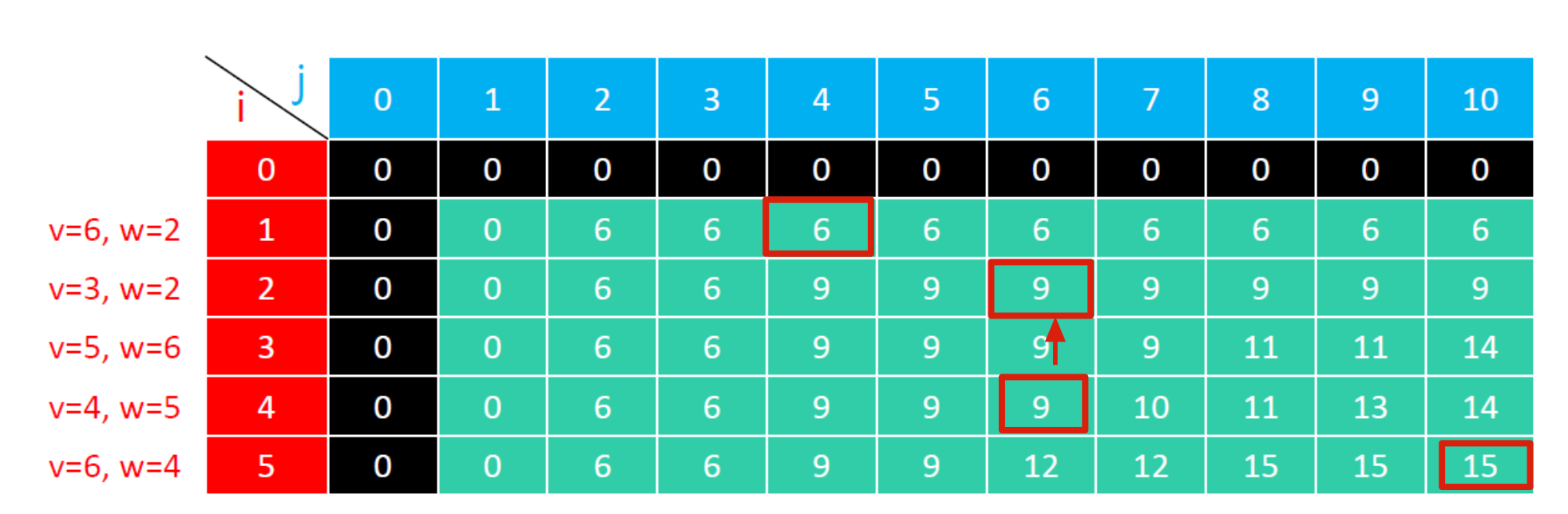• 当前列dp(i)(j) > dp(i-1)(j)
• 说明选中了物品 i，且可以反推出 dp(i,j)=values[i]+dp(i-1,j-weights[i])
• 上一个选中的物品可能是 i-1, 接下来需要判断 dp(i-1,j-weights[i]) > dp(i-2,j-weights[i]) ?
• 当前列dp(i)(j) == dp(i-1)(j) * 说明没有选中 i ，判断 dp(i-1)(j) == dp(i-2)(j) ？
``````/**
* 打印选中的商品
* @param items  完整的商品列表
* @param dp  动态规划结果表
*/
def printSelectItems(items: List[Item], dp: Array[Array[Int]]): Unit = {
var i = dp.length-1
val selectedItems = new ArrayBuffer[Item]()
while (i > 0 && j > 0) {
if (dp(i)(j) > dp(i-1)(j)) {
selectedItems += items(i-1)
j -= items(i-1).weight
}

i -= 1
}
println("selected items")
println(selectedItems.reverse.toList)
}
``````

``````// 优化数据结构； 使用 2 * J 二维数据缓存数据
def packSack2(items: List[Item], capacity: Int):Unit = {

val dp = Array.ofDim[Int](2, capacity+1)
for (i <- 1 to items.size) {
val current = i%2
val last = 1-current
val it = items(i-1)
for (j <- 1 to capacity) {
if (j < it.weight) {
dp(current)(j) = dp(last)(j)
} else {
dp(current)(j) = Math.max(dp(last)(j), dp(last)(j-it.weight)+it.value)
}
}
println(dp(current).toList)
}

println(dp.last.last)
}

// 优化数据结构； 使用 一维数据缓存数据
def packSack3(items: List[Item], capacity: Int):Unit = {

val dp = new Array[Int](capacity+1)
for (i <- 1 to items.size) {
val it = items(i-1)
// WARN: 这里需要逆序，防止数据被覆盖
for (j <- Range(capacity, it.weight-1, -1)) {
dp(j) = Math.max(dp(j), dp(j-it.weight)+it.value)
}
println(dp.toList)
}

println(dp.last)
}
``````